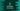# How to get the length of an array in C### How to get the length of an array in C#:

This post will show you different ways to get the length of an array in C#. To get the length or to count the total number of elements of an array, different predefined methods are available. In this post, I will show you all of these methods with examples.

### Method 1: Get the array length with Count():

The Count() method is a method of System.Linq.Enumerable class. We can use this method with any class that implements IEnumerable. We can use this method with arrays. It will return the total count of elements of the array.

``````using System;
using System.Linq;

namespace Program
{
class Program
{
static void Main(string[] args)
{
int[] Arr1 = { 1, 2, 3, 4, 5 };
string[] Arr2 = { "one", "two", "three", "four" };
bool[] Arr3 = { true, false };

Console.WriteLine("Arr1 length: " + Arr1.Count());
Console.WriteLine("Arr2 length: " + Arr2.Count());
Console.WriteLine("Arr3 length: " + Arr3.Count());
}
}
}``````

If you run this program, it will print the below result:

``````Arr1 length: 5
Arr2 length: 4
Arr3 length: 2``````

### Method 2: Count the array length of specific element types:

We can use the Count method to filter out specific elements of an array. For example, let’s filter out all elements starts with t:

``````using System;
using System.Linq;

namespace Program
{
class Program
{
static void Main(string[] args)
{
string[] Arr1 = { "one", "two", "three", "four" };

Console.WriteLine("Arr1 length: " + Arr1.Count(s => s.StartsWith("t", StringComparison.CurrentCultureIgnoreCase)));
}
}
}``````

This will return the count of all strings in Arr1 those are starts with “t”. If you run this program, it will return 2 as we have only two words starts with “t” in Arr1.

### Method 3: Length property of arrays:

The Length property of C# array also returns the total count of elements of an array. For a multi-dimension array, it returns the total number of elements in all dimensions of the array.

It’s syntax is:

``public int Length { get; }``

It returns the total number of elements in all the dimensions of the array. For an empty array, it returns 0.

It might throw OverflowException for a multidimensional array if it contains more than System.Int32.MaxValue elements.

The below example uses .Length property to find the length of an array:

``````using System;

namespace Program
{
class Program
{
static void Main(string[] args)
{
string[] Arr1 = { "one", "two", "three", "four" };

Console.WriteLine("Arr1 length: " + Arr1.Length);
}
}
}``````

This property gives the total count of elements for a multi-dimensional array. For example:

``````using System;

namespace Program
{
class Program
{
static void Main(string[] args)
{
string[,] Arr1 = new string[,] { { "one", "two", "three" }, { "hello", "world", "!!" } };

Console.WriteLine("Arr1 length: " + Arr1.Length);
}
}
}``````

It will give the total count of Arr1, which is 6.

### Method 4: By using GetLength:

The GetLength method is used to number of elements of a multi-dimensional array in a specified dimension. It returns a 32-bit integer. It takes a zero-based dimension as the parameter.

It throws IndexOutOfRangeException for an invalid dimension.

Let’s take a look at the below program:

``````using System;

namespace Program
{
class Program
{
static void Main(string[] args)
{
string[,] Arr1 = new string[,] { { "one", "two", "three" }, { "hello", "world", "!!" } };

Console.WriteLine("Arr1 GetLength(0): " + Arr1.GetLength(0));
Console.WriteLine("Arr1 GetLength(1): " + Arr1.GetLength(1));
}
}
}``````

The first line is calling GetLength with parameter 0 and the second line is calling GetLength with parameter 1.

It will print:

``````Arr1 GetLength(0): 2
Arr1 GetLength(1): 3``````